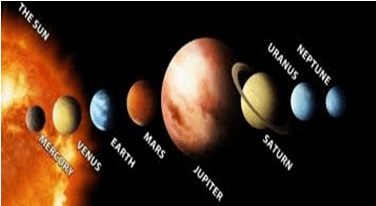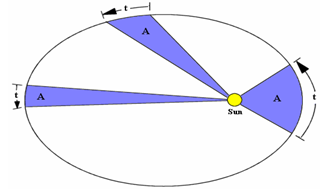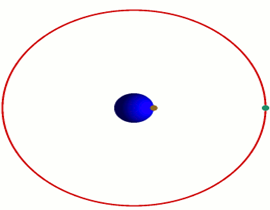Courses

# Gravitational Motion - Physics, IAS UPSC Notes | EduRev

## UPSC : Gravitational Motion - Physics, IAS UPSC Notes | EduRev

The document Gravitational Motion - Physics, IAS UPSC Notes | EduRev is a part of the UPSC Course General Science(Prelims) by IRS Divey Sethi.
All you need of UPSC at this link: UPSC

Gravitational Motion

Solar system

• It consists of sun which is stationary at the centre of the universe, a source of light and energy and eight planets (Pluto has lost it planet status) which revolve around the sun in separate orbits.
• The order of the planets in the solar system with their increasing distance from the sun is-
• Mercury
• Venus
• Earth
• Mars
• Jupiter
• Saturn
• Uranus
• Neptune• John kepler found important regularities in the motions of the planets.
• He gives three law in this context called kepler’s laws of planetary motion.

Kepler’s laws

1. all planets move around the sun in elliptical orbits having the sun at one force of the orbits.
2. The Areal speed of the planet remains constant.
3. The square of the period of revolution of any planet around the sun is directly proportional to the cube of its mean distance from the sun, i.e.,
• T2 or r3Newton’s Universal Law of Gravitation

• Every two objects in the universe attract each other.
• “The force of attraction is directly proportional to the product of masses and inversely proportional to the square of the distance between the two masses”
• F ∞ mm 2 / r²
(or) F = G . m 1 m 2 / r²
• where, G = universal gravitational constant.
• G = 6.67 x 10 -11 N m -2 kg -2

Acceleration Due to Gravity

• When a body falls freely, then the increase in velocity per second is called acceleration due to gravity.
• It is represented by g.
• g = GMe / Re²
• where, Me = mass of the earth,
• Re = radius of the earth.
• Value of g depends upon the radius of the earth as g ∞ 1 / R.
• So, value of g is maximum at poles and minimum at equator.

Variation in the Value of G With Height

• Value of g at the surface of the earth is 9.8 m / s²
• Value of g at the centre of earth is zero.
• Value of g at infinity becomes zero.
• If the body is taken inside the earth, acceleration due to gravity decreases linearly with the distance from centre of the earth.
• If the body is taken above the surface of the earth, acceleration due to gravity varies inversely as the square of the distance from the centre of earth.
• g is a vector quantity. Its direction is towards the centre of the earth.
• Its value is independent of the shape, size, mass, etc of the body but depends upon mass and radius of the earth or planet due to which there is a gravity pull.

Geostationary Satellites

• Geostationary satellites are stationary with respect to an observer on the earth.
• Their time period is 24 hrs. Their height above the surface of earth is 36,000 km.
• They are always in equatorial plane and their orbits are circular. They are also called parking orbits.
• the speed of satellite depends upon its height from the earth’s surface. The farther is the satellite from the earth’s surface, the lesser will be its speed.
• The velocity of a satellite does not depend upon its mass. There force, if various satellites of different masses revolve around the earth in same orbit then orbital velocities will be the same.• In an artificial satellite reaction of a person is zero; therefore the person does not experience any weight. This state is known as the state of weightlessness.

Offer running on EduRev: Apply code STAYHOME200 to get INR 200 off on our premium plan EduRev Infinity!

## General Science(Prelims) by IRS Divey Sethi

39 videos|110 docs|255 tests

,

,

,

,

,

,

,

,

,

,

,

,

,

,

,

,

,

,

,

,

,

,

,

,

;